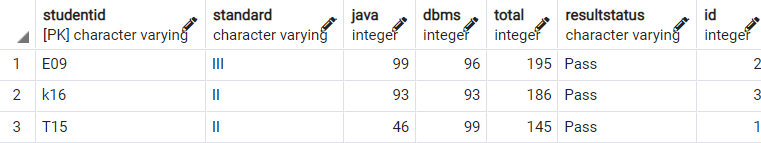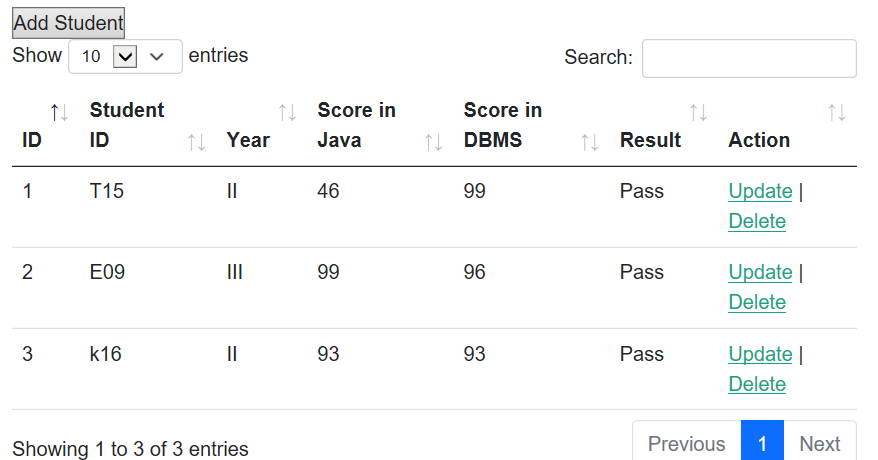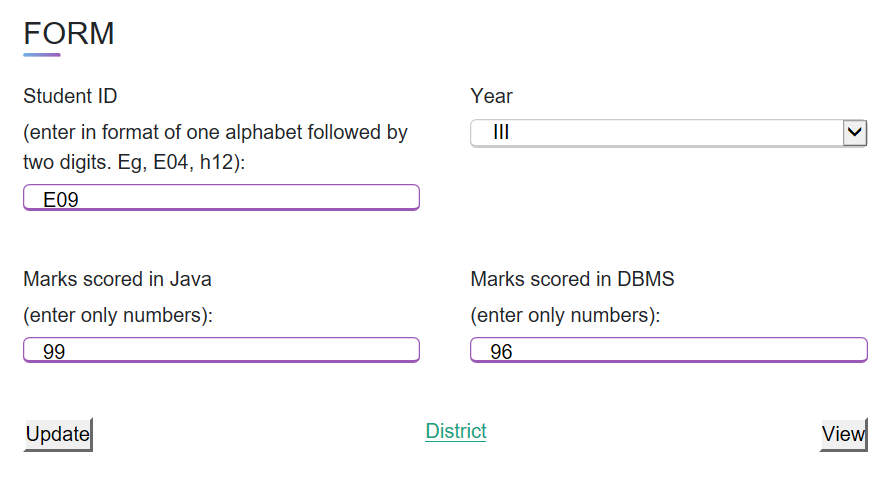# Spring – Perform Update Operation in CRUD

• Difficulty Level : Medium
• Last Updated : 06 Sep, 2022

CRUD (Create, Read, Update, Delete) operations are the building block for developers stepping into the software industry. CRUD is mostly simple and straight forward except that real-time scenarios tend to get complex. Among CRUD operations, the update operation requires more effort to get right compared to the other three operations. A pre-loaded database table is a pre-requisite for carrying out CRUD operations Read involves only reading data from the database and displaying it in the user interface (UI). Create is also similarly simple as it only involves taking input from the user and entering the validated input into the database.

Update and delete tend to get more complex to understand as it will involve the usage of data structures and other webpage concepts such as params, JSP page, etc. This tutorial aims to simplify the update operation for first-timers in CRUD. Before we get to the tutorial, it would be beneficial to see the visual representation of the operations in the web pages and databases involved in the operations.

The image given below shows the database that is to be populated on the webpage:This is how the populated table will look along with the update and delete action Buttons:Once the update button is clicked, this is how the data gets populated into the form:### Example Project

To understand how Update works, it’s necessary to start with the read operation.

## Java

 `public` `List getStudents() ``throws` `Exception {``    ``List students = ``new` `ArrayList<>();``    ``// the ArrayList students contains``      ``// all the data from the database``    ``return` `students;``}`

Now the ArrayList students now get returned to the webpage via a servlet.

Note: The actual read operation has a longer code. The code depicted here is just to throw some light on the data structures used in the create operation. The List<Student> declaration uses a class named Student with all the variables, getters, and setters to initialize the ArrayList object.

Before proceeding to the servlet, it would help a little to take a look at the object structure that would be used in this code while using ArrayLists.

Student.java

## Java

 `package` `com.jdbc;` `public` `class` `Student {``    ``private` `String name;``    ``private` `String gender;``    ``private` `String course;``    ``private` `int` `id;``    ` `    ``public` `Student(``int` `id, String name,String course, String gender) {``        ``this``.name = name;``        ``this``.gender = gender;``        ``this``.course = course;``        ``this``.id = id;``    ``}``    ` `    ``public` `String getName() {``        ``return` `name;``    ``}``    ``public` `void` `setName(String name) {``        ``this``.name = name;``    ``}``    ``public` `String getGender() {``        ``return` `gender;``    ``}``    ``public` `void` `setGender(String gender) {``        ``this``.gender = gender;``    ``}``    ``public` `String getCourse() {``        ``return` `course;``    ``}``    ``public` `void` `setCourse(String course) {``        ``this``.course = course;``    ``}``    ``public` `int` `getId() {``        ``return` `id;``    ``}``    ``public` `void` `setId(``int` `id) {``        ``this``.id = id;``    ``}``    ``@Override``    ``public` `String toString() {``        ``return` `"Student [id="` `+ id + ``", name"` `+ name + ``", course="` `+ course + ``", gender="` `+ gender + ``"]"``;``    ``}``}`

StudentControllerServlet.java

## Java

 `import` `javax.annotation.Resource;``import` `javax.servlet.RequestDispatcher;``import` `javax.servlet.ServletException;``import` `javax.servlet.annotation.WebServlet;``import` `javax.servlet.http.HttpServlet;``import` `javax.servlet.http.HttpServletRequest;``import` `javax.servlet.http.HttpServletResponse;``import` `javax.sql.DataSource;` `import` `com.jdbc.StudentDbUtil;``import` `com.jdbc.Student;` `@WebServlet``(``"/StudentControllerServlet"``)``public` `class` `StudentControllerServlet ``extends` `HttpServlet {``    ``private` `static` `final` `long` `serialVersionUID = 1L;``    ``private` `StudentDbUtil studentDbUtil;` `private` `void` `listStudents(HttpServletRequest request, HttpServletResponse response)``        ``throws` `Exception {` `        ``// get students from db util``        ``// This is where the database arraylist``          ``// is received in the servlet``        ``List students = studentDbUtil.getStudents();``        ` `        ``// add students to the request``        ``request.setAttribute(``"STUDENT_LIST"``, students);``                ` `        ``// send to JSP page (view)``        ``RequestDispatcher dispatcher =     request.getRequestDispatcher(``"/CRUDform.jsp"``);``        ` `        ``dispatcher.forward(request, response);``    ``}``}`

Code Explanation:

1. The ArrayList ‘students’ is named as STUDENT_LIST using the request.setAttribute method.
2. A dispatcher to the webpage (CRUDform.jsp) is initialized using ‘RequestDispatcher dispatcher = request.getRequestDispatcher(“/CRUDform.jsp”);’ and the control if forwarded to the same webpage using ‘dispatcher.forward(request, response);’.

CRUDform.jsp

## HTML

 `<%@ page language="java" contentType="text/html; charset=ISO-8859-1"``   ``pageEncoding="ISO-8859-1"%>``<%@ taglib uri="http://java.sun.com/jsp/jstl/core" prefix="c" %>``<%@ page import="java.util.*" %>````<``html``>``   ``<``head``>``      ``<``meta` `http-equiv``=``"Content-Type"` `content``=``"text/html; charset=UTF-8"``>``      ``<``meta` `name``=``"viewport"` `content``=``"width=device-width, initial-scale=1"``>``      ``<``meta` `http-equiv``=``"Content-Type"` `content``=``"text/html; charset=ISO-8859-1"``>``      ``<``title``>Form``   ````   ``<%``      ``// get students from the request object (sent by servlet)``      ``String crud = (String) request.getAttribute("COMMAND");``      ``if(crud == null){``        ``crud = "register";``        ``StudentControllerServlet s = new StudentControllerServlet();   ``      ``}``      ``List<``Student``> theStudent = (List<``Student``>) request.getAttribute("STUDENT_LIST");    ``      ` `      ``Student theStudents = (Student) request.getAttribute("THE_STUDENT");``      ``%>``   ``<``body``>``      ``<``table` `id``=``"example"` `class``=``"table table-striped"` `style``=``"width:100%"``>``         ``<``thead``>``            ``<``tr``>``               ``<``th``>Name``               ``<``th``>Course``               ``<``th``>Gender``            ````         ````         ``<``tbody``>``            ``<``c:forEach` `var``=``"tempStudent"` `items``=``"\${STUDENT_LIST}"``>``            ````            ``<``c:url` `var``=``"tempLink"` `value``=``"StudentControllerServlet"``>``               ``<``c:param` `name``=``"command"` `value``=``"LOAD"` `/>``               ``<``c:param` `name``=``"studentId"` `value``=``"\${tempStudent.id}"` `/>``               ``<``c:param` `name``=``"crud"` `value``=``"UPDATE"` `/>``            ````            ````            ``<``c:url` `var``=``"deleteLink"` `value``=``"StudentControllerServlet"``>``               ``<``c:param` `name``=``"command"` `value``=``"DELETE"` `/>``               ``<``c:param` `name``=``"studentId"` `value``=``"\${tempStudent.id}"` `/>``            ````            ``<``tr``>``               ``<``td``> \${tempStudent.name} ``               ``<``td``> \${tempStudent.course} ``               ``<``td``> \${tempStudent.gender} ``               ``<``td``>``                  ``<``a` `href``=``"\${tempLink}"``>Update``                  ``|``                  ``<``a` `href``=``"\${deleteLink}"``                     ``onclick``=``"if (!(confirm('Are you sure you want to delete the entry with ID \${tempStudent.studentid} and studying in \${tempStudent.standard} year?'))) return false"``>Delete   ``               ````            ````         ````      ````   `````

The above example would require the usage of JSTL tags, database so make sure to install and add the required libraries (postgresql-42.3.1.jar, javax.servlet.jsp.jstl-1.2.1.jar, and javax.servlet.jsp.jstl-api-1.2.1.jar) in the project.

Code Explanation:

1. The ArrayList with the name “STUDENT_LIST” can be retrieved using Java code written between opening braces ‘<%’ and closing braces’%>’. The command ‘(List<Student>) request.getAttribute(“STUDENT_LIST”);’ gets the attribute ‘STUDENT_LIST’ from the servlet, typecasts it into an object defined in the class name Student, and then it is stored in the variable of Object type using the command ‘List<Student> theStudent = (List<Student>) request.getAttribute(“STUDENT_LIST”);’.
2. Now the ArrayList named “theStudent” is what is used to populate tables with the database entries.
3. Here comes the usage of JSTL tags. The <c:forEach> tag, which can be considered as a JSTL equivalent of the Java “for loop”, is used to iterate through the ArrayList “STUDENT_LIST” and the tag has to be defined as follows: <c:forEach var=”tempStudent” items=”\${STUDENT_LIST}”> </c:forEach>.
4. Between the opening and closing of <c:forEach> tag comes the code for populating the table.
5. The <c:url> tag is used to format a URL into a string and store it into a variable and to perform URL rewriting when necessary. The <c:param> tag has the attributes name, value which sets the name and value of the request parameters in the URL respectively. Here, the name attribute is mandatory with no default value whereas the value parameter is not compulsory. For example, the tag ‘<c:url var=”tempLink” value=”StudentControllerServlet”><c:param name=”command” value=”LOAD” /><c:param name=”studentId” value=”\${tempStudent.id}” /><c:param name=”crud” value=”UPDATE” /></c:url>’ writes the target URL as http://localhost:8001/HibernateStudent/StudentControllerServlet?command=LOAD&studentId=k16&id=3&crud=UPDATE where the attribute command is passed with value “LOAD” which is a vital attribute.
6. When the button labeled as “UPDATE” corresponding to the LOAD attribute is pressed and the controller goes to the StudentControllerServlet mentioned in the URL given above.

StudentControllerServlet.java

Refer to this article Resource Injection in Java EE to understand the first line in the code snippet given below.

## Java

 `@Resource``(name=``"jdbc/test"``)``private` `DataSource dataSource;` `@Override``public` `void` `init() ``throws` `ServletException {``    ``super``.init();``      ` `// create our student db util ...``// and pass in the conn pool / datasource``try``{``    ``studentDbUtil = ``new` `StudentDbUtil(dataSource);``}``catch` `(Exception exc) {``    ``throw` `new` `ServletException(exc);``}` `protected` `void` `doPost(HttpServletRequest request, HttpServletResponse response) ``throws` `ServletException, IOException {``    ``try` `{``    ``String theCommand = request.getParameter(``"command"``);``        ` `    ``switch` `(theCommand) {``      ``case` `"LOAD"``:``          ``loadStudent(request, response);``        ``break``;``        ``}``    ``}``    ``catch` `(Exception exc) {``        ``throw` `new` `ServletException(exc);``    ``}``}` `private` `void` `loadStudent(HttpServletRequest request, HttpServletResponse response)``            ``throws` `Exception {``            ` `    ``// read student id from form data``    ``int` `id = Integer.parseInt(request.getParameter(``"id"``));``    ``String theStudentId = request.getParameter(``"studentId"``);``    ``String crud = request.getParameter(``"crud"``);``    ` `    ``// get student from database (db util)``    ``Student theStudent = studentDbUtil.getStudent(id);  ``            ` `    ``// get students from db util and control continues           ``    ``List students = studentDbUtil.getStudents();``            ` `    ``// add students to the request``    ``request.setAttribute(``"STUDENT_LIST"``, students);``            ` `    ``// place student in the request attribute``    ``request.setAttribute(``"THE_STUDENT"``, theStudent);``            ` `    ``request.setAttribute(``"COMMAND"``, ``"update"``);   ` `    ``// send to jsp page: CRUDform.jsp``    ``RequestDispatcher dispatcher = request.getRequestDispatcher(``"/CRUDform.jsp"``);``            ` `    ``dispatcher.forward(request, response);       ``}`

Code Explanation:

1. The annotation @Resource(name=”jdbc/test”) along with the command “private DataSource dataSource;” will be used to establish the database connection.
2. The “LOAD” command declared on the previous page is received in the servlet using the “String theCommand = request.getParameter(“command”);” command and is accordingly the control goes into the update function as per the switch case statement.
3. After entering into the loadstudent method, the attributes id, theStudentId, crud are received using requests from the previous page. Now the details corresponding to the particular ID are retrieved by invoking the “Student theStudent = studentDbUtil.getStudent(id);” by passing the ID as a parameter which is demonstrated in the code given below.

StudentDbUtil.java

## Java

 `// point of resource injection``public` `DataSource dataSource;``     ` `public` `StudentDbUtil(DataSource theDataSource) {``    ``dataSource = theDataSource;``}` `public` `Student getStudent(``int` `theStudentId) ``throws` `Exception{``        ``Student theStudent = ``null``;``        ` `        ``Connection myConn = ``null``;``        ``PreparedStatement myStmt = ``null``;``        ``ResultSet myRs = ``null``;``        ``String studentId;``        ``try` `{``            ``// get connection to database``            ``myConn = dataSource.getConnection();``            ` `            ``//create sql to get selected student``        ``String sql = ``"select * from students where id=?"``;` `        ``// create prepared statement``        ``myStmt = myConn.prepareStatement(sql);``            ` `        ``// set params``        ``myStmt.setInt(``1``, theStudentId);``            ` `        ``// execute statement``        ``myRs = myStmt.executeQuery();``            ` `        ``// retrieve data from result set row``        ``if` `(myRs.next()) {``            ``int` `id = myRs.getInt(``"id"``);``            ``String name = myRs.getString(``"name"``);``            ``String course = myRs.getString(``"course"``);``            ``String gender = myRs.getString(``"gender"``);``                ` `            ``// create new student object``            ``theStudent = ``new` `Student(id, name, course, gender);``        ``}``        ``else` `{``            ``throw` `new` `Exception(``"Could not find student id: "` `+ theStudentId);``        ``}   ``        ``return` `theStudent;``        ``}``        ``finally` `{``            ``close(myConn, myStmt, myRs);``        ``}``    ``}`

Code Explanation:

1. The line ‘studentDbUtil.getStudent(id);’ invokes the corresponding method in the StudentDbUtil.java class.
2. The command ‘myConn = dataSource.getConnection();’ gets a connection to the database. After this command, the ‘myConn’ variable will be used to reference the database connectivity wherever required.
3. After that, in the try block, the select query ‘String sql = “select * from students where id=?”;’, the prepared statement ‘myStmt = myConn.prepareStatement(sql);’ are declared and parameters are set for retrieving the corresponding data.
4. The id is set in the query using ‘myStmt.setInt(1, theStudentId);’ and the select query is executed with ‘myRs = myStmt.executeQuery();’ and stored in the result set (myRs) variable.
5. The result set is iterated and an object is invoked to store the data which is to be passed to the webpage.
6. The connection is closed in the finally block and now it’s time to return the control to the servlet.

## Java

 `private` `void` `loadStudent(HttpServletRequest request, HttpServletResponse response)``            ``throws` `Exception {                                                             ``            ` `    ``// get students from db util and control continues           ``    ``List students = studentDbUtil.getStudents();``            ` `    ``// add students to the request``    ``request.setAttribute(``"STUDENT_LIST"``, students);``            ` `    ``// place student in the request attribute. This is what is used to pre-populate the form with data from the database.``    ``request.setAttribute(``"THE_STUDENT"``, theStudent);``    ` `    ``// the attribute command is set to update for identification purpose.       ``    ``request.setAttribute(``"COMMAND"``, ``"update"``);   ` `    ``// send to jsp page: CRUDform.jsp``    ``RequestDispatcher dispatcher = request.getRequestDispatcher(``"/CRUDform.jsp"``);``            ` `    ``dispatcher.forward(request, response);       ``}`

The control gets directed to CRUDform.jsp page and the attributes are checked within the ‘<%’ and ‘%>’ tags before the body tags to decide whether it is an update or list, or register operation.

## HTML

 `<%``   ``// get students from the request object (sent by servlet)``   ``String crud = (String) request.getAttribute("COMMAND");``   ``if(crud == null){``       ``crud = "register";``       ``StudentControllerServlet s = new StudentControllerServlet();   ``   ``}``   ` `   ``List<``Student``> theStudent = (List<``Student``>) request.getAttribute("STUDENT_LIST");    ``   ` `   ``Student theStudents = (Student) request.getAttribute("THE_STUDENT");``   ``%>``<``body``>``   ``<``div` `class``=``"container"``>``   ``<``div` `class``=``"title"``>FORM``   ``<``div` `class``=``"content"``>``   ``<``form` `name``=``"myForm"` `action``=``"StudentControllerServlet"` `method``=``"POST"` `onsubmit``=``"return validateForm()"``>``      ``<%``         ``if(crud.equals("register")){%>``      ``<``input` `type``=``"hidden"` `name``=``"command"` `value``=``"ADD"` `/>``      ``<%}else if(crud.equals("update")){%>``      ``<``input` `type``=``"hidden"` `name``=``"command"` `value``=``"UPDATE"` `/>``      ``<``input` `type``=``"hidden"` `name``=``"id"` `value``=``"\${THE_STUDENT.id}"` `/>``      ``<%}``         ``%>``      ``<``div` `class``=``"user-details"``>``      ``<``div` `class``=``"input-box"``>``         ``<``span` `class``=``"details"``>Name ``         ``<``input` `type``=``"text"` `name``=``"name"` `placeholder``=``"Enter name"` `required ``value``=``"\${THE_STUDENT.name}"``/> <``br``><``br``>``      ````      ``<``div` `class``=``"input-box"``>``         ``<``span` `class``=``"details"``>Course ``         ``<``select` `name``=``"course"` `id``=``"course"``>``            ``<%if(crud.equals("register")){%>``            ``<``option` `value``=``"CSE"``>CSE``            ``<``option` `value``=``"IT"``>IT``            ``<``option` `value``=``"ECE"``>ECE``            ``<``br``><``br``>``         ````         ``<%}else if(crud.equals("update")){%>``         ``<%if(theStudents.getCourse().equals("CSE")){%>``         ``<``option` `value``=``"CSE"` `selected>CSE``         ``<%}else{%>``         ``<``option` `value``=``"CSE"``>CSE``         ``<%}%>``         ``<%if(theStudents.getCourse().equals("IT")){%>``         ``<``option` `value``=``"IT"` `selected>IT``         ``<%}else{%>``         ``<``option` `value``=``"IT"``>IT``         ``<%}%>``         ``<%if(theStudents.getCourse().equals("ECE")){%>``         ``<``option` `value``=``"ECE"` `selected>ECE``         ``<%}else{%>``         ``<``option` `value``=``"ECE"``>ECE``         ``<%}%>``         ``<%}%>``         ````         ``<%if(crud.equals("register")){%>``         ``<``input` `type``=``"radio"` `id``=``"male"` `name``=``"gender"` `value``=``"Male"``> ``         ``<``label` `for``=``"male"``>Male ``         ``<``label` `for``=``"male"``>Female ``         ``<``label` `for``=``"male"``>Transgender ``         ````         ``<%}else if(crud.equals("update")){%>``         ``<%if(theStudents.getGender().equals("Male")){%>``         ``<``input` `type``=``"radio"` `id``=``"male"` `name``=``"gender"` `value``=``"Male"` `checked> ``         ``<``label` `for``=``"male"``>Male ``         ``<%}else{%>``         ``<``input` `type``=``"radio"` `id``=``"male"` `name``=``"gender"` `value``=``"Male"``> ``         ``<``label` `for``=``"male"``>Male ``         ``<%}%>``         ``<%if(theStudents.getGender().equals("Female")){%>``         ``<``input` `type``=``"radio"` `id``=``"female"` `name``=``"gender"` `value``=``"Female"` `checked> ``         ``<``label` `for``=``"female"``>Female ``         ``<%}else{%>``         ``<``input` `type``=``"radio"` `id``=``"female"` `name``=``"gender"` `value``=``"Female"``> ``         ``<``label` `for``=``"female"``>Female ``         ``<%}%>``         ``<%if(theStudents.getGender().equals("Transgender")){%>``         ``<``input` `type``=``"radio"` `id``=``"transgender"` `name``=``"gender"` `value``=``"Transgender"` `checked> ``         ``<``label` `for``=``"transgender"``>Transgender ``         ``<%}else{%>``         ``<``input` `type``=``"radio"` `id``=``"transgender"` `name``=``"gender"` `value``=``"Transgender"``> ``         ``<``label` `for``=``"transgender"``>Transgender ``         ``<%}%>``         ``<%}%>``      ````      ``<%``         ``if(crud.equals("update")){%>``      ``<``button` `name``=``"submit"` `value``=``"SUBMIT"` `type``=``"submit"``>Update``      ``<%}else if(crud.equals("register")){%>``      ``<``button` `name``=``"submit"` `value``=``"SUBMIT"` `type``=``"submit"``>Register``      ``<%}%>       ``   ```

Code Explanation:

1. The attribute “COMMAND” is received in the JSP page using ‘String crud = (String) request.getAttribute(“COMMAND”);’ and stored in the variable named ‘crud’.
2. The ArrayLists ‘List<Student> theStudent = (List<Student>) request.getAttribute(“STUDENT_LIST”);’ and ‘Student theStudents = (Student) request.getAttribute(“THE_STUDENT”);’ are received from the servlet.
3. The value of ‘crud’ is checked and if it has the value “update” then a hidden input type is initiated to indicate that the operation is an “update” operation when the form is submitted.
4. Auto populating a text or date picker field is straightforward enough, adding value=”\${THE_STUDENT.name}” to the corresponding tag will do the work. Here, THE_STUDENT denotes the ArrayList name, name denotes the ‘name variable’ in the ArrayList and \${} is used since it’s Java code being used in HTML.
5. Now comes the real challenge for beginners, the value attribute does not work in dropdown or radio buttons. This is where JSTL tags come to save the day.
6. Condition checking is done and components are auto selected accordingly. For example, the command <%if(theStudents.getCourse().equals(“CSE”)){%> checks if the value of course in the ArrayList is equal to CSE and if the condition is satisfied then the option CSE is auto selected in the dropdown using <option value=”CSE” selected>CSE</option>. If not, then the else clause is executed where the HTML tag is entered without being selected <%}else{%> <option value=”CSE”>CSE</option> <%}%>
7. The above if and else in JSTL format can also be applied for radio buttons for auto-selecting according to the data in the database only change is the usage of “checked” keyword instead of selected as demonstrated in the code given above.
8. The control goes to the servlet code given below when the form is submitted.

StudentControllerServlet.java

## Java

 `protected` `void` `doPost(HttpServletRequest request, HttpServletResponse response) ``throws` `ServletException, IOException {``        ``try` `{``            ``// read the "command" parameter``            ``String theCommand = request.getParameter(``"command"``);``            ` `            ``// route to the appropriate method``            ``switch` `(theCommand) {``                ` `            ``case` `"UPDATE"``:``            ``updateStudent(request, response);``            ``break``;``                ` `            ``}``        ``catch` `(Exception exc) {``            ``throw` `new` `ServletException(exc);``        ``}``        ` `    ``}` `private` `void` `updateStudent(HttpServletRequest request, HttpServletResponse response)``            ``throws` `Exception {``            ``// read student info from form data``            ``int` `id = Integer.parseInt(request.getParameter(``"id"``));``            ``String name = request.getParameter(``"name"``);``            ``String course = request.getParameter(``"course"``);``            ``String gender = request.getParameter(``"gender"``);``            ``// create a new student object``            ``Student theStudent = ``new` `Student(id,name,course,gender);``            ` `            ``// perform update on database``            ``studentDbUtil.updateStudent(theStudent);``            ` `            ``// get students from db util``            ``List students = studentDbUtil.getStudents();``            ` `            ``// add students to the request``            ``request.setAttribute(``"STUDENT_LIST"``, students);``        ` `            ``// send them back to the "list students" page``            ``listStudents(request, response);``    ``}`

As demonstrated in the previous servlet example, the control goes to the database class in the line ‘studentDbUtil.updateStudent(theStudent);’

StudentDbUtil.java

## Java

 `public` `void` `updateStudent(Student theStudent) ``throws` `Exception{``        ``Connection myConn = ``null``;``        ``PreparedStatement myStmt = ``null``;``        ` `        ``try` `{``            ``// get connection to database``            ``myConn = dataSource.getConnection();``            ` `            ``// create SQL update statement``            ``String sql = ``"update student "``                    ``+ ``"set  name=?,gender=?,course=? "``                    ``+ ``"where id=?"``;``            ` `            ``// prepare statement``            ``myStmt = myConn.prepareStatement(sql);``            ` `            ``//set params``            ``myStmt.setString(``1``, theStudent.getName());``            ``myStmt.setString(``2``, theStudent.getGender());``            ``myStmt.setString(``3``, theStudent.getCourse());``            ``myStmt.setInt(``4``, theStudent.getId());   ``    ` `            ``myStmt.execute();``        ``}``        ``finally` `{``            ``// clean up JDBC objects``            ``close(myConn, myStmt, ``null``);``        ``}``    ``}`

Now the connection is established, the query is written and the query is executed in the code given above. Update is the comparatively harder part of CRUD operations, especially populating radio buttons and dropdown lists and it has been simplified to a large extent in this article. So, we’re done now!

Note: It is highly recommended to create a SERIAL column named ‘id’ when creating the table and then use that unrelated column in the WHERE clause of SQL queries so as to avoid errors while executing queries.

My Personal Notes arrow_drop_up